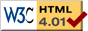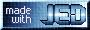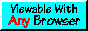# SLxfig Plotting Examples

On this page, you will find examples of some plots and drawings that were created using the SLxfig module. Click on a figure below to see the code that generated it.

A group at the Dr. Karl Remeis observatory of the University of Erlangen-Nuremberg has also compiled a very nice set of SLxfig examples.

 This is a simple plot of a damped exponential.This plot illustrates the use of color. Note the ``small-caps'' font used in the caption.A shaded histogram.Here are two stacked plots sharing a common X axis.This plot contains both X and Y errorbarsThis image plot uses S-Lang's drywet colormap.This plot shows the ratios of the speed of the python Numeric and numarray extensions to speed of S-Lang as a function of array size. As the plot shows, S-Lang is about 10 times faster than numarray is for small arrays, and almost twice as fast for large arrays. It also shows the well-known fact that numarray is worse than Numeric for small arrays. The dramatic decrease in speed of numarray for arrays larger than 10000 elements may be due to cache misses.This so-called order-sorting plot shows an image of a binned Chandra level 1.5 event list. The X axis corresponds to the Chandra MEG grating wavelength and the Y axis corresponds to an effective grating order given as the product of the grating wavelength and the ACIS CCD energy. Such a plot is extremely useful for diagnosing problems with the order-sorting process as well as the gain calibration of the CCDs.I created this figure to illustrate the features that one might see in an order-sorting plot.Plots of this type were created for a paper on the Con-X off-plane gratings. This example provides a simple illustration of the vbox/hbox statcking functions.This figure was created to graphically illustrate the meaning of some of the maplib module's projections. Did I mention that SLxfig can do 3-d projections?The bottom plot shows, among other things, the effect of a proposed sub-pixel algorithm for ACIS data.This page was last updated Nov 26, 2017 by John E. Davis. To comment on it or the material presented here, send email to jed at jedsoft org.